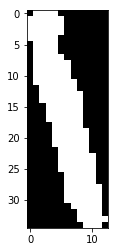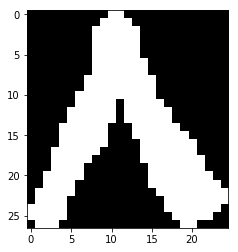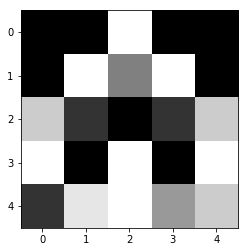به نام خدا

# معرفی مجموعه داده هدی و تغییر اندازه و شکل برای استفاده در الگوریتم‌های یادگیری ماشین

## معرفی مجموعه داده ارقام دستنویس هدی¶

مجموعه ارقام دستنویس هدی که اولین مجموعه‌ی بزرگ ارقام دستنویس فارسی است، مشتمل بر ۱۰۲۳۵۳ نمونه دستنوشته سیاه سفید است. این مجموعه طی انجام یک پروژه‏ی کارشناسی ارشد درباره بازشناسی فرمهای دستنویس تهیه شده است. داده های این مجموعه از حدود ۱۲۰۰۰ فرم ثبت نام آزمون سراسری کارشناسی ارشد سال ۱۳۸۴ و آزمون کاردانی پیوسته‏ی دانشگاه جامع علمی کاربردی سال ۱۳۸۳ استخراج شده است.

## لود کتابخانه‌های مورد استفاده¶

برای اجرای این نوت‌بوک باید کتابخانه‌های numpy و scipy و matplotlib و scikit-image را نصب کنید. بدین منظور به اینترنت متصل شود و در cmd دستورات زیر را بنویسید:

pip install numpy

pip install scipy

pip install matplotlib

pip install scikit-image

میتوانید به جای pip از کلمه ی conda استفاده کنید. (اگر از آناکوندا استفاده میکنید.)
In :
import numpy as np
from scipy import io
import matplotlib.pyplot as plt
from skimage.transform import resize


## لود مجموعه داده (dataset)¶

این مجموعه داده در قالب فایل mat متلب منتشر شده است. در پایتون میتوانیم آن را با کتابخانه scipy لود کنیم.
In :
#load dataset


## انتخاب مجموعه داده آموزش و مجموعه داده آزمون(تست)¶

در این مجموعه داده 60000 داده تصادفی وجود دارد.
برای آزمایشاتمان 1000 داده‌ی اول را برای آموزش و 200 داده‌ی بعدی را برای آزمون انتخاب می‌کنیم.
**داده های آزمون نباید قبلا در داده های آموزشی دیده شده باشند.**
In :
#test and training set
X_train_orginal = np.squeeze(dataset['Data'][:1000])
y_train = np.squeeze(dataset['labels'][:1000])
X_test_original = np.squeeze(dataset['Data'][1000:1200])
y_test = np.squeeze(dataset['labels'][1000:1200])


"squeeze": Remove single-dimensional entries from the shape of an array.

نگاهی به مجموعه داده بیندازیم!
In :
plt.imshow(X_train_orginal, cmap='gray')
plt.show()In :
i=70
image = X_train_orginal[i]
label = y_train[i]
plt.imshow(image, cmap='gray')
plt.show()
print("Label {}th image is: {}".format(i, label))Label 70th image is: 8

در کد بالا مقدار i را تغییر دهید و تصاویر را ببینید

## تغییر اندازه تصاویر مجموعه داده¶

اندازه تصاویر مجموعه داده متفاوت است.
تمام تصاویر را به یک اندازه مشخص (5*5) تغییر اندازه می‌دهیم.
In :
#resize
X_train_5by5 = [resize(img, (5, 5)) for img in X_train_orginal]
X_test_5by_5 = [resize(img, (5, 5)) for img in X_test_original]

C:\Users\alire\Miniconda3\envs\tensorflow\lib\site-packages\skimage\transform\_warps.py:105: UserWarning: The default mode, 'constant', will be changed to 'reflect' in skimage 0.15.
warn("The default mode, 'constant', will be changed to 'reflect' in "
C:\Users\alire\Miniconda3\envs\tensorflow\lib\site-packages\skimage\transform\_warps.py:110: UserWarning: Anti-aliasing will be enabled by default in skimage 0.15 to avoid aliasing artifacts when down-sampling images.
warn("Anti-aliasing will be enabled by default in skimage 0.15 to "

In :
image = X_train_5by5
plt.imshow(image, cmap='gray')
plt.show()## تغییر شکل مجموعه داده¶

حالا داده های تصویری در مقیاس 5x5 است. برای استفاده در الگوریتم‌های خود آن ها را به یک بردار 25 تایی تغییر شکل میدهیم.
In :
#reshape
X_train = [x.reshape(25) for x in X_train_5by5]
X_test = [x.reshape(25) for x in X_test_5by_5]

In :
X_train.shape

Out:
(25,)
دوره مقدماتی یادگیری عمیق
علیرضا اخوان پور
پنج شنبه، ۱۸ بهمن ۱۳۹۷
Class.Vision - AkhavanPour.ir - GitHub
In [ ]: